# PTC Thermistors (POSISTOR) Application Guide of Thermistors

- Application Example of Thermistors -

## Basic Characteristics

POSISTOR has three main characteristics

1. Resistance - Temperature Characteristics

POSISTOR has three main characteristics.
Although there is a negligible difference between the normal and "Curie Point" temperature, POSISTOR shows almost constant resistance-temperature characteristics. Yet they have resistance-temperature characteristics that cause resistance to sharply increase when the temperature exceeds the Curie Point.
The Curie Point (C.P.) is defined as the temperature at which the resistance value is twice the one at 25°C.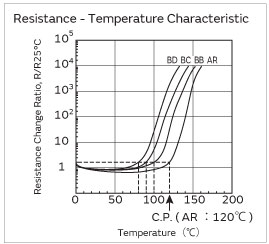2. Current - Voltage Characteristics (Static Characteristic)

This shows the relation between applied voltage when voltage applied to POSISTOR causes balancing of inner heating and outer thermal dissipation and stabilized current. This has both a maximum point of current and constant output power.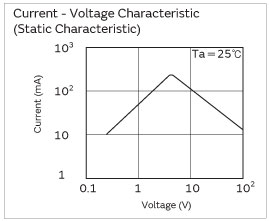3. Current - Time Characteristics (Dynamic Characteristic)

This shows the relation between current and time before inner heating and outer thermal dissipation arrive at equilibrium state. This features having large initial current and abruptly continuous attenuating portion.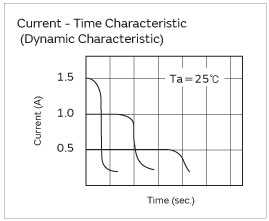## Technical Terms

1. Protective Threshold Current

The maximum current value is called the "Protective Threshold Current" for Voltage vs. Current characteristics(static).
When smaller than the protective threshold current flows in POSISTOR, it reaches its stability (as shown in figure on right) at the intersection (A) of the load curve (a) and voltage-current characteristics of POSISTOR(c). And POSISTOR works as a normal fixed resistor.
However, when larger than protective threshold current flows, it stabilizes at the intersection (B) with the load curve (b).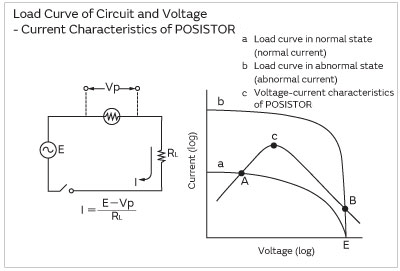Figure 1

2. Protective Threshold Current Range

Protective threshold current varies depending on the ambient temperature, resistance value, temperature characteristics and shape. (see Figure 2) The maximum value of trip current and the minimum value of the hold current are in the range of ambient temperature -10 to +60°C.
That is, when a current is smaller than the hold current, POSISTOR works only as a fixed resistor. When larger than the trip current flows, however, POSISTOR protects the circuit from overload.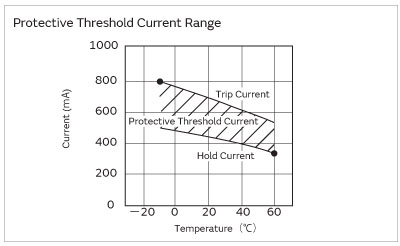Figure 2

3. Operating Time

A period starting from the voltage input to the moment current itself sharply attenuates is called "Operating Time." Conventionally, operation time (t0) is determined to be the period until inrush current (I0) decreases to a level one half the original inrush current (I0/2).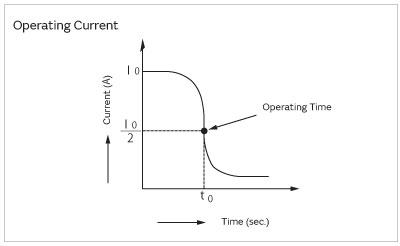Figure 3